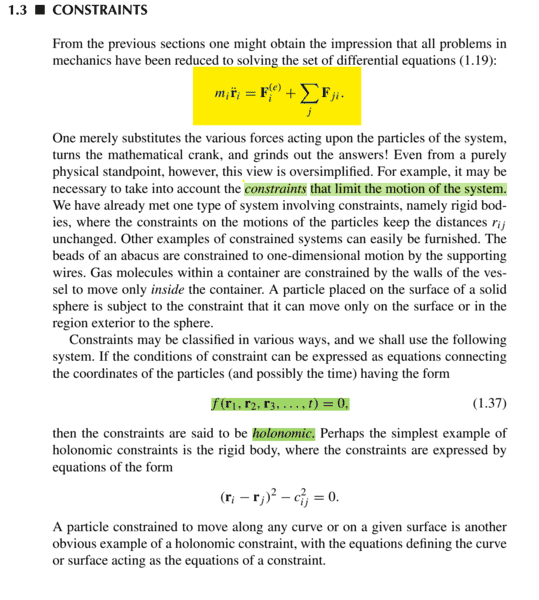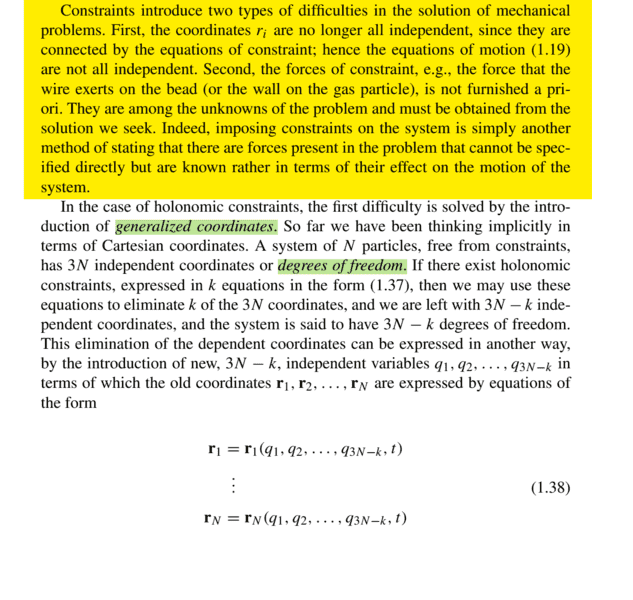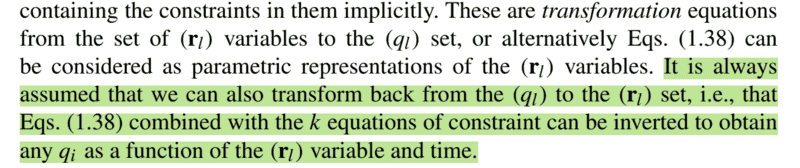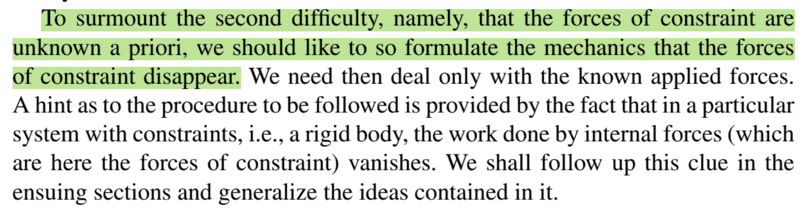# Why do we need the Lagrangian formulation of Mechanics?

• Pushoam
In summary, Lagrangian formulation of mechanics allows us to solve equations of motion without knowing the forces acting on the particle. This is a difficulty because the position coordinates are connected by equations of constraint. However, generalized coordinates can be used to remove this difficulty.f

#### PushoamThese images have been taken from Goldstein, Classical Mechanics.
Why do we need Lagrangian formulation of mechanics when we already have Newtonian formulation of mechanics?
Newtonian formulation of mechanics demands us to solve the equation of motion given by equation 1. 19. for this we need to know all the forces acting on the particle. But in general there exists forces which are unknown to us but their effects on the system is known to us. These forces are known as constraint forces. The effects of these forces are known as constraints and and these effects are expressed in terms of equations.

Thus constraint forces introduce two types of difficulties : 1) these forces are an mom so we cannot solve the equations of motions directly. Hence we need a formulation in which we do not need to know the constraint forces.
2) the position coordinates are connected by equations of constraint and hence, these are no more linearly independent.
But, how can this be a difficulty? We can express linearly dependent coordinates in terms of linearly independent coordinates and then solve equations for linearly independent coordinates and then substitute the values of linearly independent coordinates to find the linearly dependent coordinates. Hence, how can this be a difficulty and why do we need generalized coordinates to remove it?

#### Attachments

Last edited:
We can express linearly dependent coordinates in terms of linearly independent coordinates and then solve equations for linearly independent coordinates and then substitute the values of linearly independent coordinates to find the linearly dependent coordinates. Hence, how can this be a difficulty and why do we need generalized coordinates to remove it?
How would these linearly independent coordinates be different from the generalized coordinates?

•Dale
Why do we need Lagrangian formulation of mechanics when we already have Newtonian formulation of mechanics?
Why do we need light switches when we can just screw light bulbs in?

It makes things easier.

•shihab-kol, Pushoam and DrClaude
For me it's the opposite. As soon as I learned analytical mechanics, I came to the question why we were bothered with "naive" mechanics, particularly with forces, which usually are not as easy to analyze as writing down the Lagrangian in the appropriate coordinates and then just calculate.

One warning: In newer editions of Goldstein the anholonomous constraints are treated in a wrong way. Rather use Landau Lifshitz vol. I, when you want to learn it or stick to d'Alembert's principle, which gives the correct equations for anholonomous constraints.

•Pushoam and weirdoguy
Why do we need light switches when we can just screw light bulbs in?

It makes things easier.
That's because light bulbs are not allowed anymore (at least not in the EU) but only LEDs ;-)).

•shihab-kol, Cryo, DrClaude and 1 other person
@Pushoam It's also worth noting that the Lagrangian and Hamiltonian formulations generalise to GR and QM; whereas, the concept of a force does not.

•Cryo, Pushoam, DrClaude and 1 other person
There is always a cost benefit trade one needs to do. For example, the upside of quaternions is they provide computational advantages to computing rotations in three dimensions. The down side is one needs to learn quaternions. In the end it boils down to do quaternions make you happy.

Thanks to all of you.
We can express linearly dependent coordinates in terms of linearly independent coordinates and then solve equations for linearly independent coordinates and then substitute the values of linearly independent coordinates to find the linearly dependent coordinates. Hence, how can this be a difficulty and why do we need generalized coordinates to remove it?
How would these linearly independent coordinates be different from the generalized coordinates?
When I said coordinates, I meant position coordinates. I saw it different from generalized coordinates as generalized coordinates need not be position coordinates in all cases.
Now, I see that by definition, linearly independent position coordinates are also generalized coordinates. Generalized coordinates are general version of position coordinates.

In addition to what everyone else has said, there is a big advantage to using Lagrangians as opposed to starting with equations of motion: If you put in an interaction between two subsystems in a Lagrangian, it automatically enforces a generalization of Newton's rule about equal and opposite forces. If subsystem A affects subsystem B, then subsystem B affects subsystem A in just the right way so that momentum is conserved.

When you use Lagrangians to describe the interaction between a particle and a field, the same interaction term describes both the effect of the field on the particle and the effect of the particle on the field, so that automatically (for example) a particle that is influenced by an electromagnetic field can serve as a source for an electromagnetic field.

It's tricky to make this work out if you try to just jump straight to the equations of motion.

•Dale, vanhees71, Cryo and 2 others